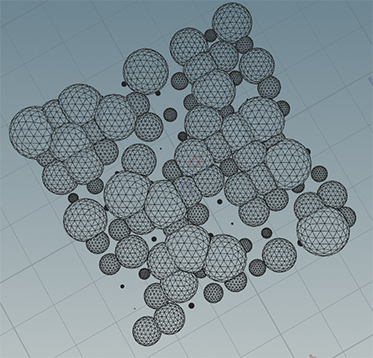# I need a simple way to fix geometries intersections.

## Recommended Posts

Hi guys,

I'm looking for a (simple and fast) way to fix intersections between geometries. I found this video:

But the problem is the result is not that satisfying.

I would appreciate any ideas.

Thanks for helping.

##### Share on other sites

Quote

But the problem is the result is not that satisfying.

This doesn't give us any information about you issue, show your "not satisfying" result, share setup or example...
The setup from the video is working as expected, tested.

•1
##### Share on other sites

Have Some points and pscale att on those use this after

```//get neighbour pts finding a arrary
int nPts[] = nearpoints(geoself(), @P, chf("maxdist"), chi("maxpts"));
pop(nPts, 0);
i[]@nPts = nPts;
i@id = @ptnum;
matrix mArray[];
float targetD[];

foreach(int pt; nPts){
float nRadius = point(geoself(), "pscale", pt);
vector nPos = point(geoself(), "P", pt);
vector myPos = @P;
float distance = distance(nPos,myPos);

if ( distance < collideLength){
//intersectNums += 1;
//set localMatrix[] by nearPoints
vector z = normalize(nPos - myPos);
vector x = normalize( cross(set(0,1,0), z));
vector y = cross(z, x);
matrix m = set(x,y,z, myPos);
m.xw = 0.0;
m.yw = 0.0;
m.zw = 0.0;
append(mArray, m);

//calculate intersect distance
float intersectD = (pow(myRadius, 2) - pow(nRadius, 2) + pow(distance, 2)) / (2.0 * distance);
append(targetD, intersectD);
}
}
4[]@mArray = mArray;
f[]@targetD = targetD;```

then copy to points and than use this

```//foreach neighbour pts and check if the point sphere intersect with another guy
int nPts[] = i[]@nPts;
float targetD[] = point(1, "targetD", i@id);
matrix mArray[] = point(1, "mArray", i@id);

//set deform weight
float weight = 0;
foreach(int i; int pt; nPts){

//calculate intersect distance
float intersectD = targetD[i];
//check if the Pt Pos > myRadius
matrix m = mArray[i];
vector newPos = @P * invert(m);
if( newPos.z > intersectD){
vector pos = newPos;
pos = set(pos.x, pos.y, intersectD);
@P = pos * m;
weight = 1;
}
//weight = fit(newPos.z, -f@pscale, intersectD + chf("weight_offset"), 0, 1);

}
//f@weight = weight;
f@weight = chramp("weight_ramp", weight);```

then blur weight Play with Code and Setup...##### Share on other sites

On 12/29/2022 at 8:30 AM, Librarian said:

Have Some points and pscale att on those use this after

```//get neighbour pts finding a arrary
int nPts[] = nearpoints(geoself(), @P, chf("maxdist"), chi("maxpts"));
pop(nPts, 0);
i[]@nPts = nPts;
i@id = @ptnum;
matrix mArray[];
float targetD[];

foreach(int pt; nPts){
float nRadius = point(geoself(), "pscale", pt);
vector nPos = point(geoself(), "P", pt);
vector myPos = @P;
float distance = distance(nPos,myPos);

if ( distance < collideLength){
//intersectNums += 1;
//set localMatrix[] by nearPoints
vector z = normalize(nPos - myPos);
vector x = normalize( cross(set(0,1,0), z));
vector y = cross(z, x);
matrix m = set(x,y,z, myPos);
m.xw = 0.0;
m.yw = 0.0;
m.zw = 0.0;
append(mArray, m);

//calculate intersect distance
float intersectD = (pow(myRadius, 2) - pow(nRadius, 2) + pow(distance, 2)) / (2.0 * distance);
append(targetD, intersectD);
}
}
4[]@mArray = mArray;
f[]@targetD = targetD;```

then copy to points and than use this

```//foreach neighbour pts and check if the point sphere intersect with another guy
int nPts[] = i[]@nPts;
float targetD[] = point(1, "targetD", i@id);
matrix mArray[] = point(1, "mArray", i@id);

//set deform weight
float weight = 0;
foreach(int i; int pt; nPts){

//calculate intersect distance
float intersectD = targetD[i];
//check if the Pt Pos > myRadius
matrix m = mArray[i];
vector newPos = @P * invert(m);
if( newPos.z > intersectD){
vector pos = newPos;
pos = set(pos.x, pos.y, intersectD);
@P = pos * m;
weight = 1;
}
//weight = fit(newPos.z, -f@pscale, intersectD + chf("weight_offset"), 0, 1);

}
//f@weight = weight;
f@weight = chramp("weight_ramp", weight);```

then blur weight Play with Code and Setup...

Can you post scene. This code doesnt work for me.

Thanks

## Join the conversation

You can post now and register later. If you have an account, sign in now to post with your account.
Note: Your post will require moderator approval before it will be visible.×   Pasted as rich text.   Paste as plain text instead

Only 75 emoji are allowed.

×   Your previous content has been restored.   Clear editor

×   You cannot paste images directly. Upload or insert images from URL.

×
×